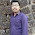## Friday, June 24, 2011

### SQL: Turn multiple rows into one row multiple columns

We have a table looks like this:
Name Question Value
Alan        1           5
Alan        2           4
Alan        3           6
Jim          1           4
Jim          2           3
Jim          4           5

We would like to select out a result looks like this:
Name    Q1     Q2    Q3    Q4
Alan       5        4       6      null
Jim          4       3       null   5

This problem seems to be very easy, however, cost me a lot of time to figure out how to proceed. Finally, after some googling a nice post introduce a nice way to handle similar issues using MAX(DECODE()) function.

Basically, you first need to utilise the decode() function. decode() acts like if-else-then. For example, decode(name, 'Alan', 'True', 'False') works like if the name is 'Alan' then return 'True', otherwise return 'False'.

In our problem here, we use decode() to distinguish the answers from different questions: decode(question, 1, value, NULL) as Q1,   decode(question, 2, value, NULL) as Q2, ... In this way we would pick out the value of each question.

After we can get the answer to each question, what we need to do is group the answers by different names. That's why we need max() here to act for the group by clause. Since there is only one value here for each question, so max(), min() or some other reasonable functions are all working well here.:

select name AS NAME, max(decode(question, 1, value, NULL)) AS Q1, max(decode(question, 2, value, NULL)) AS Q2, max(decode(question, 3, value, NULL)) AS Q3, max(decode(question, 4, value, NULL)) AS Q4 from table group by name.

1.2.